Courses

# Legendre Special Function (Part - 5) - Mathematical Methods of Physics, UGC - NET Physics Physics Notes | EduRev

## Physics for IIT JAM, UGC - NET, CSIR NET

Created by: Akhilesh Thakur

## Physics : Legendre Special Function (Part - 5) - Mathematical Methods of Physics, UGC - NET Physics Physics Notes | EduRev

The document Legendre Special Function (Part - 5) - Mathematical Methods of Physics, UGC - NET Physics Physics Notes | EduRev is a part of the Physics Course Physics for IIT JAM, UGC - NET, CSIR NET.
All you need of Physics at this link: Physics

Example 12.5.3 MAGNETIC INDUCTION FIELD OF A CURRENT LOOP

Like the other ODEs of mathematical physics, the associated Legendre equation is likely to pop up quite unexpectedly. As an illustration, consider the magnetic induction ﬁeld B and magnetic vector potential A created by a single circular current loop in the equatorial plane (Fig. 12.12).
We know from electromagnetic theory that the contribution of current element Id λ to the magnetic vector potential is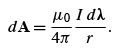(12.113)
(This follows from Exercise 1.14.4 and Section 9.7) Equation (12.113), plus the symmetry of our system, shows that A has only a ϕˆ component and that the component is independent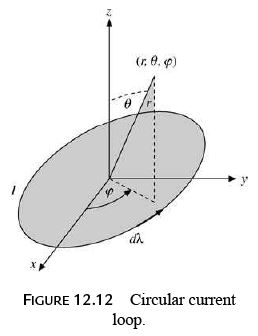of ϕ ,17

A = ϕˆ Aϕ (r, θ ).             (12.114)

By Maxwell’s equations,

∇ × H = J,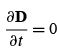(SI units).              (12.115)

Since

µ0H = B = ∇ × A,           (12.116)

we have

∇ × (∇ × A) = µ0J,               (12.117)

where J is the current density. In our problem J is zero everywhere except in the current loop. Therefore, away from the loop,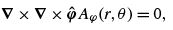(12.118)

using Eq. (12.114).

From the expression for the curl in spherical polar coordinates (Section 2.5), we obtain (Example 2.5.2)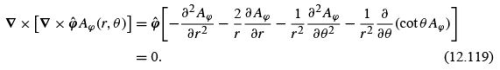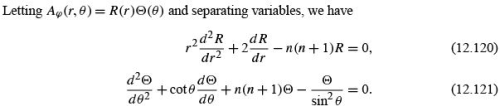The second equation is the associated Legendre equation (12.80) with m = 1, and we may immediately write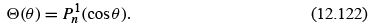The separation constant n(n + 1), n a nonnegative integer, was chosen to keep this solution well behaved.
By trial, letting R(r ) = r α , we ﬁnd that α = n,or −n − 1. The ﬁrst possibility is discarded, for our solution must vanish as r →∞. Hence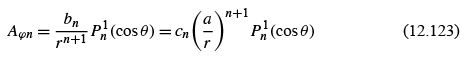and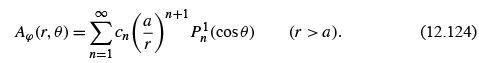Here a is the radius of the current loop.
Since Aϕ must be invariant to reﬂection in the equatorial plane, by the symmetry of our problem,

Aϕ (r, cos θ) = Aϕ (r, − cos θ),                   (12.125)

the parity property of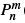(cos θ) (Eq. (12.97)) shows that cn = 0 for n even.
To complete the evaluation of the constants, we may use Eq. (12.124) to calculate Bz along the z-axis (Bz = Br (r, θ = 0)) and compare with the expression obtained from the Biot–Savart law. This is the same technique as used in Example 12.3.3. We have (compare Eq. (2.47))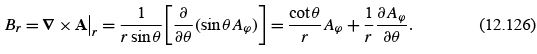Using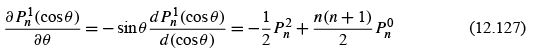(Eq. (12.94)) and then Eq. (12.91) with m = 1,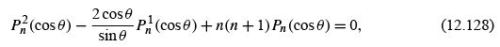we obtain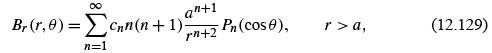(for all θ ). In particular, for θ = 0,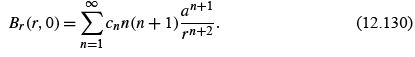We may also obtain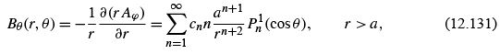The Biot–Savart law states that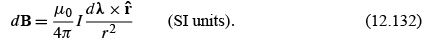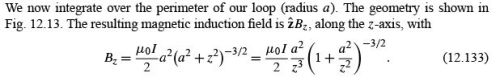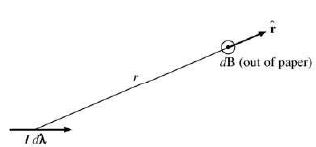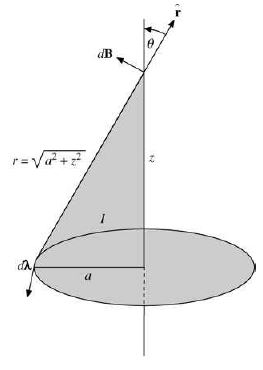FIGURE 12.13 Biot–Savart law applied to a circular loop.

Expanding by the binomial theorem, we obtain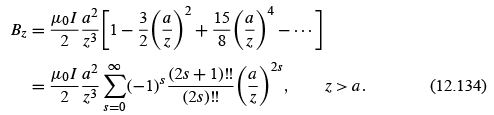Equating Eqs. (12.130) and (12.134) term by term (with r = z),18 we ﬁnd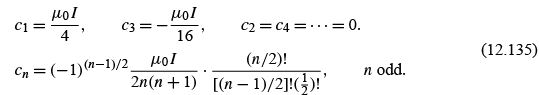Equivalently, we may write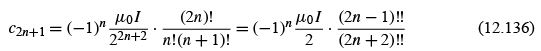and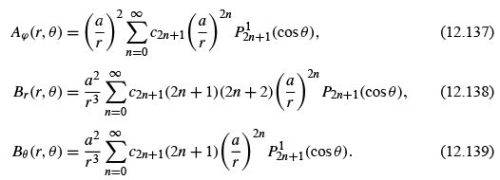These ﬁelds may be described in closed form by the use of elliptic integrals. Exercise 5.8.4 is an illustration of this approach. A third possibility is direct integration of Eq. (12.113) by expanding the denominator of the integral for Aϕ in Exercise 5.8.4 as a Legendre polynomial generating function. The current is speciﬁed by Dirac delta functions. These methods have the advantage of yielding the constants cn directly.
A comparison of magnetic current loop dipole ﬁelds and ﬁnite electric dipole ﬁelds may be of interest. For the magnetic current loop dipole, the preceding analysis gives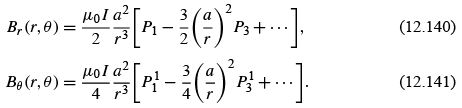From the ﬁnite electric dipole potential of Section 12.1 we have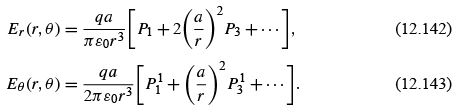The two ﬁelds agree in form as far as the leading term is concerned (r −3 P1 ), and this is the basis for calling them both dipole ﬁelds.
As with electric multipoles, it is sometimes convenient to discuss point magnetic multipoles (see Fig. 12.14). For the dipole case, Eqs. (12.140) and (12.141), the point dipole is formed by taking the limit a → 0,I →∞, with Iaheld constant. With n a unit vector normal to the current loop (positive sense by right-hand rule, Section 1.10), the magnetic moment m is given by m = nIπ a2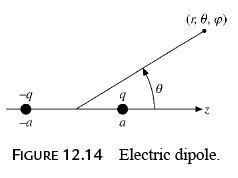SPHERICAL HARMONICS

In the separation of variables of (1) Laplace’s equation, (2) Helmholtz’s or the spacedependence of the electromagnetic wave equation, and (3) the Schrödinger wave equation for central force ﬁelds,

2 ψ + k2 f(r) ψ = 0,                      (12.144)

the angular dependence, coming entirely from the Laplacian operator, is19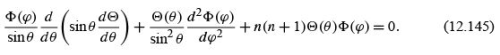Azimuthal Dependence — Orthogonality

The separated azimuthal equation is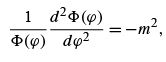(12.146)

with solutions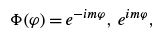(12.147)

with m integer, which satisfy the orthogonal condition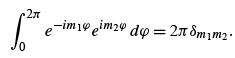(12.148)

Notice that it is the product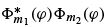that is taken and that ∗ is used to indicate the complex conjugate function. This choice is not required, but it is convenient for quantum mechanical calculations. We could have used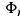= sin mϕ , cos mϕ                 (12.149)

and the conditions of orthogonality that form the basis for Fourier series .
For applications such as describing the Earth’s gravitational or magnetic ﬁeld, sin mϕ and cos mϕ would be the preferred choice (see Example 12.6.1).
In electrostatics and most other physical problems we require m to be an integer in order that(ϕ ) be a single-valued function of the azimuth angle. In quantum mechanics the question is much more involved: Compare the footnote in Section 9.3.
By means of Eq. (12.148),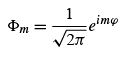(12.150)

is orthonormal (orthogonal and normalized) with respect to integration over the azimuth angle ϕ .

Offer running on EduRev: Apply code STAYHOME200 to get INR 200 off on our premium plan EduRev Infinity!

159 docs

,

,

,

,

,

,

,

,

,

,

,

,

,

,

,

,

,

,

,

,

,

,

,

,

;